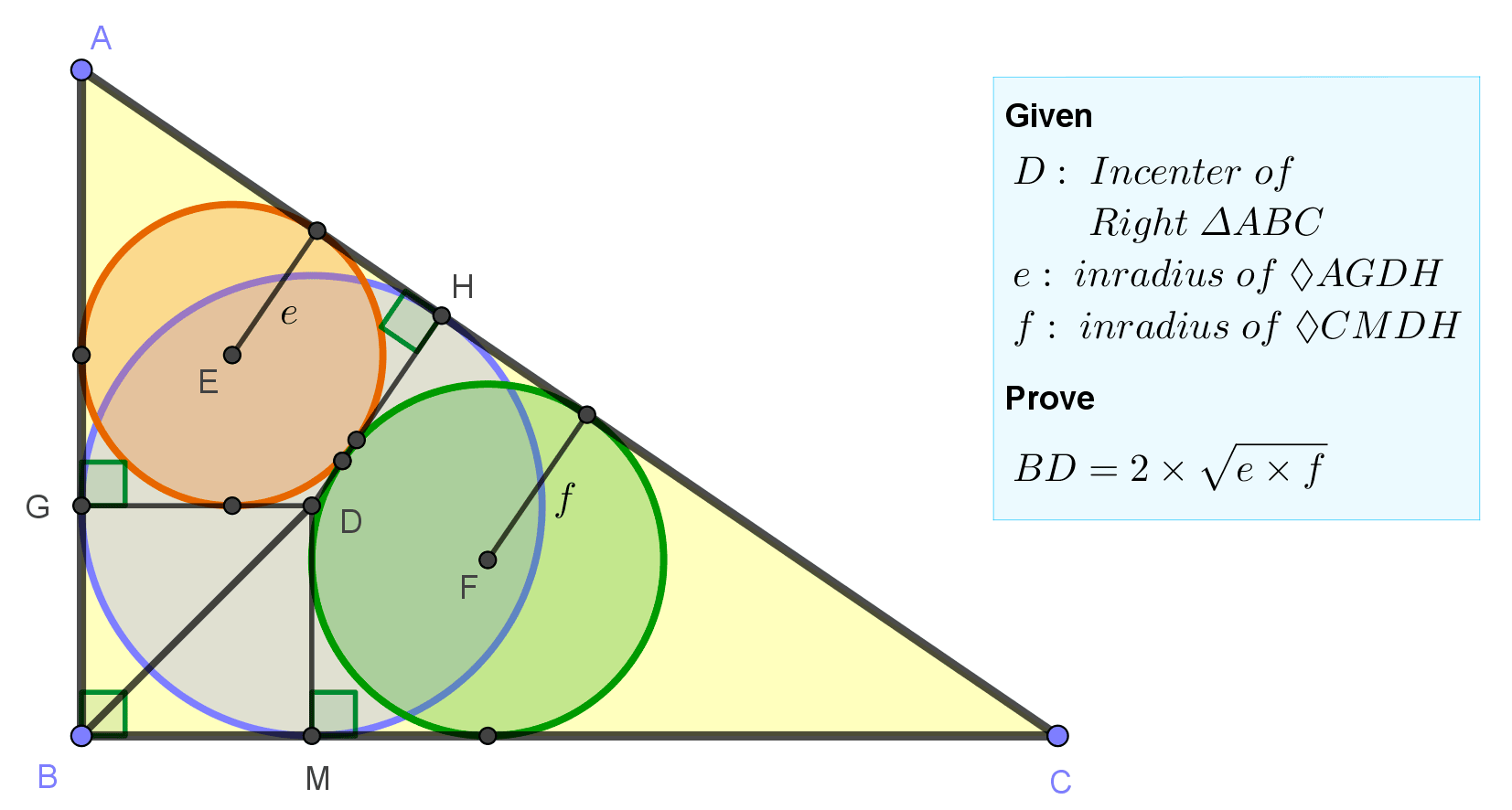# Geometry Problem 1502: Right Triangle, Incircle, Inradius, Geometric Mean of Two Inradii, Angle Bisector, Perpendicular, and Tangential Quadrilateral

In the figure, D is the incenter of right triangle ABC, $$e$$ and $$f$$ are the inradii of the tangential quadrilaterals AGDH and CMDH, respectively. Prove that $$BD=2\cdot\sqrt {e\cdot f}$$